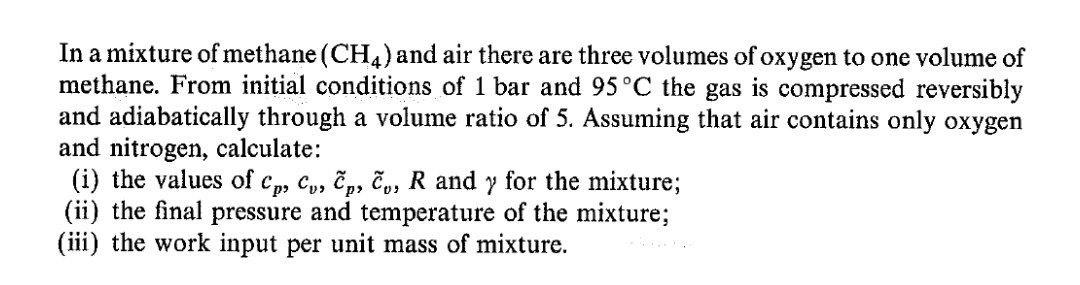Home / Expert Answers / Chemical Engineering / in-a-mixture-of-methane-left-mathrm-ch-4-right-and-air-there-are-three-volumes-of-oxyge-pa691

# (Solved): In a mixture of methane $$\left(\mathrm{CH}_{4}\right)$$ and air there are three volumes of oxyge ...In a mixture of methane $$\left(\mathrm{CH}_{4}\right)$$ and air there are three volumes of oxygen to one volume of methane. From initial conditions of 1 bar and $$95^{\circ} \mathrm{C}$$ the gas is compressed reversibly and adiabatically through a volume ratio of 5 . Assuming that air contains only oxygen and nitrogen, calculate: (i) the values of $$c_{p}, c_{v}, \tilde{c}_{p}, \tilde{c}_{v}, R$$ and $$\gamma$$ for the mixture; (ii) the final pressure and temperature of the mixture; (iii) the work input per unit mass of mixture.

We have an Answer from Expert